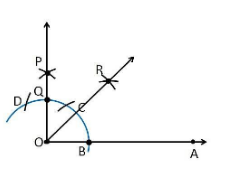# Construct an angle of 45° at the initial point of a given ray and justify the construction.Procedure

To construct an angle of 45°, the given steps can be followed

1. Let us draw a ray OA

2. Considering O as a centre with any radius, draw an arc DCB that cuts OA at B.

3.Considering B as a centre with the same radius, mark a point C on the arc DCB.

4. Considering C as a centre and the same radius, mark a point D on the arc DCB.

5. Now let us take C and D as the centre, draw two arcs that intersect each other with the same radius at P.

6. Now the ray OP is joined which makes an angle of 90° with OP is formed.

7. Considering B and Q as centre let us draw the perpendicular bisector which intersects at the point R

8. Draw a line that joins the point O and R

9. So, the angle formed ∠ROA = 45°

Justification

From the construction,

∠POA = 90°

From the perpendicular bisector from the point B and Q, which divides the ∠POA into two halves. So it becomes

∠ROA = ½ ∠POA

∠ROA = (½)×90° = 45°

Hence Proved